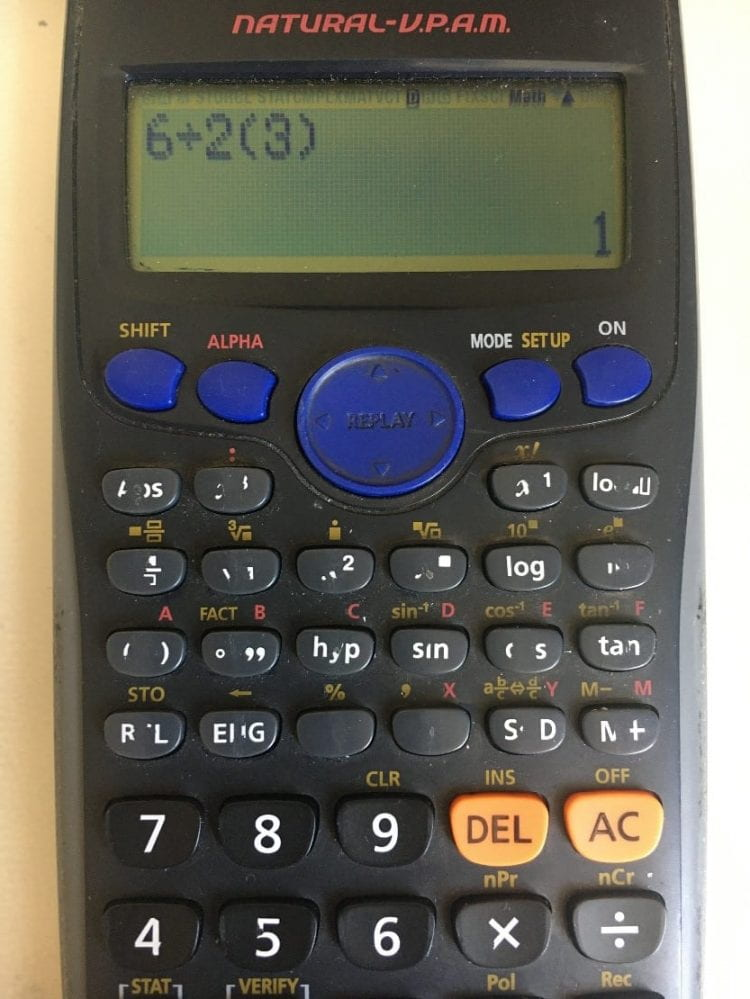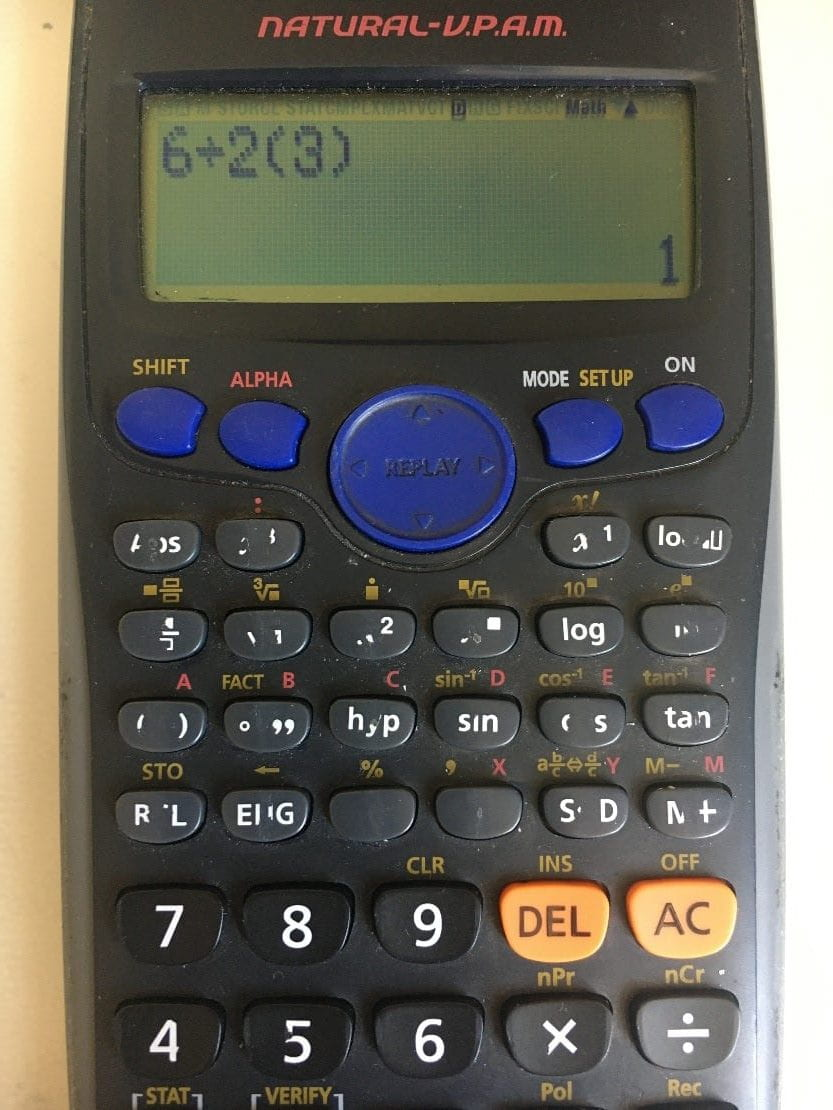# Maths blog: operation orders and the history behind it

6÷2(1+2) =? What is the value of this mathematical expression?

This question was taken from a year 5 maths problem in the chapter of operation. This question has gone viral on the internet and received millions of comments on social media sites. The problem is an example of order of operations. These are referred to as ‘BODMAS’ this means the order of operation should be carried out in the order of: Brackets, orders, multiplication-division and finally addition and subtraction. If the operation has the same precedence, the calculation is then carried out from left to right.

Using these rules, we can see the expression simplifies to 6÷2(3). At this stage where the debate online begins. A correct way solves this is to carry out the division first as division and multiplication has the same precedence. So, it simplifies further down to 3 multiplied by 3 which is 9. Any modern scientific calculator will provide this answer.

However, if you type this into an older calculator, we can get another widely debated answer: 1. As we can see on my older calculator I used for my GCSE.I found some historical documents that did have a slightly different understanding of the division symbol in different texts. Reference: http://www.math.ucdenver.edu/~jloats/Student%20pdfs/4_Order%20of%20OperationsSass.pdf

The first part of the operation is still the same, we still carry out the operation in the brackets first. When we arrive at the most debated stage of 6÷2(3). It turns out in 1907 or before, this used to mean the entire product in the left divided by the entire product in the right. So, in the historical perspective, the expression 6÷2(3) equals to 6/2(3). Of course, in that case the expression would be equals to 1 which is outputted by my older calculator.

You might be wondering why did they used to do that? Some of the research show that the printing technology they have back then have limited vertical printing capabilities, this greatly prohibited the printing of the fraction sign, where it will required the numerator to be printed on top of the denominator. So back in 1907 or before, books used the division symbol instead. When they wrote x÷2y, they meant x/2y.

However today, we don’t use this practice it can be very confusing and impractical, if you want to divide an expression you need to use the fraction symbol.

So, the correct answer to the question is 9, but there is some historical justification to the answer 1. Unfortunately for those for argued for this answer, it is wrong in today’s standard.